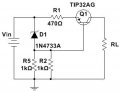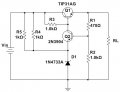# Help with identifying these circuits.

#### Wolframore

Joined Jan 21, 2019
1,318
Can someone help me identify the biasing and operation of these amplifier circuits? We went from basics voltage divider, base or emitter bias and feedback to this on a lab. It looks like a voltage divider bias with common emitter using a Zener to set the bias. I’m not sure why R5 and R2 is in parallel could have just used a 470...This looks like a CE Q2 controlling a emitter follower Q1... please confirm#### ericgibbs

Joined Jan 29, 2010
8,860
hi W,
They are simple Voltage regulator circuits.
E

•Wolframore

#### bertus

Joined Apr 5, 2008
20,101
Hello,

The upper circuit looks like a constant current source to me.
The value will be about (5.1-0.7)/470 = 9.36 mA

The second circuit is a simple voltage regulator.
The voltage on the base of Q2 is kept constant at about 5.1+0.7 Volts = 5.8 Volts
The output voltage will be 5.8 * (R1+R2)/R2 = 8.53 Volts.

Bertus

#### Jony130

Joined Feb 17, 2009
5,007
The first one looks more like a constant current source.

The second one is a voltage regulator. With a output voltage equal to around Vout = (5.1V + Vbe_Q2)*(1 + R1/R2) = 8.5V
And Q1 is a series pass transistor (emitter follower) and Q2 transistor (error amplifier CE amp) conducts when the base voltage is higher than the Zener voltage 5.1V plus Vbe_Q2 diode drop. When it conducts, it steals the current from the Q1 base node (R3 current enters Q2 reducing the Q1 base current) thus limiting the voltage, which acts as voltage regulation. And the Zener diode (D1) provides the reference voltage for Q1 and R4, R5 bias the Zener diode.
More here
•